EOQ

The optimal volume and frequency of orders required to satisfy a given level of demand

What is EOQ?

EOQ stands for Economic Order Quantity. It is a measurement used in the field of Operations, Logistics, and Supply Management. In essence, EOQ is a tool used to determine the volume and frequency of orders required to satisfy a given level of demand while minimizing the cost per order.

The Importance of EOQ

The Economic Order Quantity is a set point designed to help companies minimize the cost of ordering and holding inventory. The cost of ordering an inventory falls with the increase in ordering volume due to purchasing on economies of scale. However, as the size of inventory grows, the cost of holding the inventory rises. EOQ is the exact point that minimizes both these inversely related costs.

EOQ Formula

The Economic Order Quantity formula is calculated by minimizing the total cost per order by setting the first order derivative to zero. The components of the formula that make up the total cost per order are the cost of holding inventory and the cost of ordering that inventory. The key notations in understanding the EOQ formula are as follows:

Components of the EOQ Formula:

D: Annual Quantity Demanded

Q: Volume per Order

S: Ordering Cost (Fixed Cost)

C: Unit Cost (Variable Cost)

H: Holding Cost (Variable Cost)

i: Carrying Cost (Interest Rate)

Ordering Cost

The number of orders that occur annually can be found by dividing the annual demand by the volume per order. The formula can be expressed as: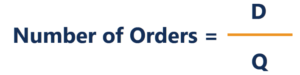For each order with a fixed cost that is independent of the number of units, S, the annual ordering cost is found by multiplying the number of orders by this fixed cost. It is expressed as:

Holding Cost

Holding inventory often comes with its own costs. This cost can be in the form of direct costs incurred by financing the storage of said inventory or the opportunity cost of holding inventory instead of investing the money tied up in inventory elsewhere. As such, the holding cost per unit is often expressed as the cost per unit multiplied by the interest rate, expressed as follows:

H = iC

With the assumption that demand is constant, the quantity of stock can be seen to be depleting at a constant rate over time. When inventory reaches zero, an order is placed and replenishes inventory as shown: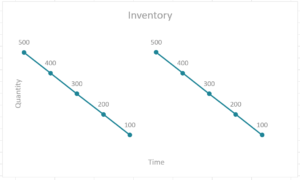As such, the holding cost of the inventory is calculated by finding the sum product of the inventory at any instant and the holding cost per unit. It is expressed as follows: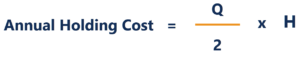Total Cost and the Economic Order Quantity

Summing the two costs together gives the annual total cost of orders. To find the optimal quantity that minimizes this cost, the annual total cost is differentiated with respect to Q. It is shown as follows: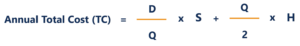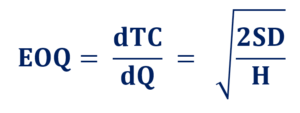Example

For example, a company faces an annual demand of 2,000 units. It costs the company \$1,000 for every order placed and \$250 per unit of the product. It faces a carrying cost of 10% of a unit cost. What is the economic order quantity?

The variables can be arranged as follows: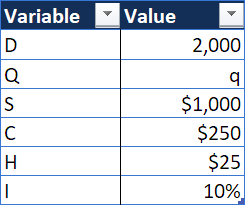The cost for each value of Q is shown as: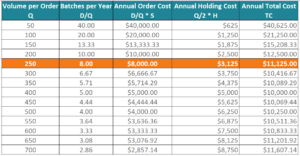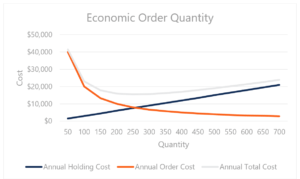Enter your name and email in the form below and download the free template now!

Other Resources

• Law of Supply
• Economies of Scope
• Consumer Surplus Formula
• Financial Analyst Program

Financial Analyst Training

Get world-class financial training with CFI’s online certified financial analyst training program!

Gain the confidence you need to move up the ladder in a high powered corporate finance career path.

Learn financial modeling and valuation in Excel the easy way, with step-by-step training.